# JP2005201869A - Signal-processing method, signal-processing program, recording medium with the program stored, and signal processing apparatus - Google Patents

Signal-processing method, signal-processing program, recording medium with the program stored, and signal processing apparatus Download PDF

## Info

Publication number
JP2005201869A
JP2005201869A JP2004010918A JP2004010918A JP2005201869A JP 2005201869 A JP2005201869 A JP 2005201869A JP 2004010918 A JP2004010918 A JP 2004010918A JP 2004010918 A JP2004010918 A JP 2004010918A JP 2005201869 A JP2005201869 A JP 2005201869A
Authority
JP
Japan
Prior art keywords
weight
measurement data
spline
signal processing
step
Prior art date
Legal status (The legal status is an assumption and is not a legal conclusion. Google has not performed a legal analysis and makes no representation as to the accuracy of the status listed.)
Withdrawn
Application number
JP2004010918A
Other languages
Japanese (ja)
Inventor
Tomonori Goto
Kozo Umeda

Original Assignee
Mitsutoyo Corp

Priority date (The priority date is an assumption and is not a legal conclusion. Google has not performed a legal analysis and makes no representation as to the accuracy of the date listed.)
Filing date
Publication date
Application filed by Mitsutoyo Corp, 株式会社ミツトヨ filed Critical Mitsutoyo Corp
Priority to JP2004010918A priority Critical patent/JP2005201869A/en
Publication of JP2005201869A publication Critical patent/JP2005201869A/en
Application status is Withdrawn legal-status Critical

## Images

•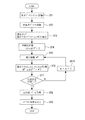•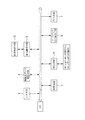•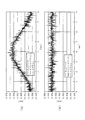••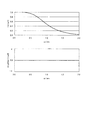•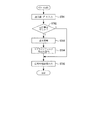•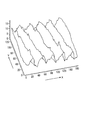•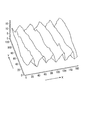•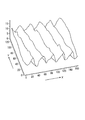••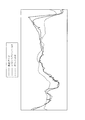•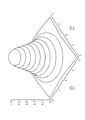•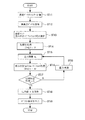## Classifications

• GPHYSICS
• G01MEASURING; TESTING
• G01DMEASURING NOT SPECIALLY ADAPTED FOR A SPECIFIC VARIABLE; ARRANGEMENTS FOR MEASURING TWO OR MORE VARIABLES NOT COVERED IN A SINGLE OTHER SUBCLASS; TARIFF METERING APPARATUS; MEASURING OR TESTING NOT OTHERWISE PROVIDED FOR
• G01D1/00Measuring arrangements giving results other than momentary value of variable, of general application
• GPHYSICS
• G01MEASURING; TESTING
• G01BMEASURING LENGTH, THICKNESS OR SIMILAR LINEAR DIMENSIONS; MEASURING ANGLES; MEASURING AREAS; MEASURING IRREGULARITIES OF SURFACES OR CONTOURS
• G01B21/00Measuring arrangements or details thereof in so far as they are not adapted to particular types of measuring means of the preceding groups
• G01B21/02Measuring arrangements or details thereof in so far as they are not adapted to particular types of measuring means of the preceding groups for measuring length, width, or thickness
• G01B21/04Measuring arrangements or details thereof in so far as they are not adapted to particular types of measuring means of the preceding groups for measuring length, width, or thickness by measuring coordinates of points
• G01B21/045Correction of measurements
• GPHYSICS
• G01MEASURING; TESTING
• G01DMEASURING NOT SPECIALLY ADAPTED FOR A SPECIFIC VARIABLE; ARRANGEMENTS FOR MEASURING TWO OR MORE VARIABLES NOT COVERED IN A SINGLE OTHER SUBCLASS; TARIFF METERING APPARATUS; MEASURING OR TESTING NOT OTHERWISE PROVIDED FOR
• G01D3/00Indicating or recording apparatus with provision for the special purposes referred to in the subgroups
• G01D3/02Indicating or recording apparatus with provision for the special purposes referred to in the subgroups with provision for altering or correcting the law of variation
• G01D3/022Indicating or recording apparatus with provision for the special purposes referred to in the subgroups with provision for altering or correcting the law of variation having an ideal characteristic, map or correction data stored in a digital memory
• GPHYSICS
• G06COMPUTING; CALCULATING; COUNTING
• G06TIMAGE DATA PROCESSING OR GENERATION, IN GENERAL
• G06T5/00Image enhancement or restoration
• G06T5/001Image restoration
• G06T5/002Denoising; Smoothing

## Abstract

<P>PROBLEM TO BE SOLVED: To provide a signal-processing method for robust spline filtering processing. <P>SOLUTION: After measurement data are inputted, a weighted spline expression is selected to determine the initial value of a spline filter. Then a weight is adjusted to compute the output of the spline filter. Then convergence determination is performed. When the weight is determined as being non-converging, the weight is updated. The weight adjustment and the computation of the spline filter output are repeated, to perform robust spline filtering on the measurement data in the signal-processing method. <P>COPYRIGHT: (C)2005,JPO&NCIPI

## Description

The present invention relates to a signal processing method, and more particularly, to a signal processing method for performing filter processing on data obtained by measuring the surface roughness, surface shape, and the like of an object to be measured.

A three-dimensional measuring machine for measuring the three-dimensional shape of the object to be measured, a contour shape measuring machine and an image measuring machine for measuring a two-dimensional contour shape, a roundness measuring machine for measuring roundness, and a surface of the object to be measured. 2. Description of the Related Art A surface texture measuring instrument that measures the contour shape, roughness, undulation, etc. of the surface of an object to be measured, such as a surface roughness measuring instrument that measures swell and roughness, is known. These collect the measurement data of the surface of the object to be measured by relatively moving the contact type or non-contact type sensor and the object to be measured.

The measurement data collected in this way usually includes disturbance components such as noise.
As the disturbance component, there are many electrical and magnetic induction noises including high-frequency components. For example, when it is desired to obtain the contour shape of the surface of the object to be measured, components such as surface roughness and swell can be disturbance components.
In order to remove such disturbance components as necessary, the measurement data is filtered to remove, for example, high-frequency components. A spline filter is known as such a filter (Patent Documents 1 and 2). Further, a robust spline filter is known in which each measurement data is weighted and the weight is updated until the weighting becomes optimum.

Japanese Patent Laid-Open No. 9-179992 JP-A-8-278343

However, the robust spline filter can be applied to curve data, but cannot be applied to curved surface data obtained by measuring a surface area.

The present invention has been made to solve the above problems, and can be applied to data obtained by measurement of a surface area while retaining the characteristics of a spline filter, and surface measurement data such as the surface properties of the object to be measured. It is an object of the present invention to provide a highly reliable signal processing method that can be applied to the present invention, and to provide a signal processing program, a recording medium on which the signal processing program is recorded, and a signal processing apparatus.

The present invention relates to a signal processing method for filtering measurement data obtained by measuring a surface area, a measurement data input step for inputting measurement data, and a selection step for selecting a predetermined weighted spline filter type. An initialization step of giving a weight for the measurement data in a unit matrix to obtain an initial value of a spline filter output, a weight adjustment step for adjusting and determining a weight for the measurement data, and a weight adjustment step A spline filter output calculating step for obtaining a spline filter output using a weight; a convergence determining step for determining convergence of the weight; and an output step for outputting a signal processing result based on the spline filter output, the convergence determination If it is determined in the step that the weight is non-convergent, the weight The weight adjustment step and the spline filter output calculation step are repeated, a smoothed spline curved surface corresponding to the measurement data is generated, and a robust spline filter process is performed on the measurement data. And

According to the present invention, a weighted spline filter equation is selected, the spline curved surface that is the spline filter output is repeatedly calculated while the weight is sequentially updated based on the selected spline filter equation, and the weight is converged. Robust spline filter processing using the spline curved surface as a filter output as a signal processing result can be easily performed on the measurement data. Therefore, it is possible to prevent the end-effect at the start point of the measurement data or the end point region of the measurement data, and the shape included in the measurement data without being affected by the follow-up characteristic or the noise component of the long period included in the measurement data. Can be extracted. As a result, it is possible to perform filter processing with good shape following characteristics, and the reliability of measurement data is further improved.

Here, the measurement data obtained by measuring the surface area means, for example, data obtained by scanning the surface of the object to be measured with a three-dimensional measuring machine or a surface texture measuring machine. That is, it means data represented by height data f (x, y) from the surface with respect to the sampling position (x, y).

In the present invention, it is preferable that the weight determined in the weight adjusting step is adjusted to be smaller as the distance of the measurement data from the spline curved surface calculated by the weighted spline filter equation is larger.

According to this invention, the larger the distance of the measurement data from the spline curved surface calculated by the weighted spline filter equation, the smaller the weight, so that the robust spline filter processing that is not affected by abnormal data included in the measurement data Is possible. In other words, the weight of the measurement data away from the spline curved surface is small, the weight of the measurement data close to the spline curved surface is increased, and the spline curved surface is obtained repeatedly. Then, the spline curved surface gradually approaches the original shape component (for example, the shape true value of the object to be measured) included in the measurement data. The last spline curved surface at the time when it is determined that the weight has converged is obtained as a shape component having a sufficiently small error with respect to the original shape component. As a result, extremely good robust spline filter processing can be performed.

In the present invention, it is preferable that the convergence determination step determines that the weight has converged when a change in the weight determined in the weight adjustment step becomes a predetermined value or less.

According to the present invention, it is possible to determine that the weight has converged when the change in the weight in the iterative loop processing is equal to or less than a predetermined value. Processing time can be shortened. Furthermore, since it can be determined that the error with the original shape component included in the measurement data is sufficiently small for the spline curved surface when the change in weight is equal to or less than a predetermined value, extremely good robust spline filter processing can be performed. It will be.

In the present invention, the output step includes a weight update step for updating the weight to 1 when the weight for the measurement data exceeds a predetermined value, and a spline filter for obtaining a spline filter output based on the updated weight. It is preferable to include a re-output calculation step and a signal processing result output step of outputting the spline filter output in the spline filter re-output calculation step as a signal processing result.

According to the present invention, when the weight at the time when it is determined that the weight has converged in the convergence determination step exceeds the predetermined value, the weight is updated to 1. When the weight is less than the predetermined value, the weight is updated to 0. Then, the spline filter output can be obtained again and the result can be output as the signal processing result. That is, when it is determined that the weight has converged by repeating the weight adjustment step and the spline filter output calculation step, the measurement data at the point where the weight exceeds a predetermined value is regarded as valid data and the weight is updated to 1. The measurement data when the weight is equal to or less than the predetermined value is regarded as invalid, and the weight is updated to 0, and then the spline filter output is obtained again. Then, the robust spline filter calculation for the measurement data can be performed more reliably. Since the result is output as a signal processing result, a spline curved surface having a sufficiently small error with respect to the original shape component included in the measurement data can be obtained. As a result, it is possible to perform robust spline filtering with good shape tracking.

In the present invention, it is preferable that the measurement data input step includes a step of deleting locally separated singularity data with respect to the measurement data.

According to the present invention, for example, due to generation of strong inductive noise using a power device in a factory or the like as a noise source, locally protruding and separated data included in measurement data (for example, data on both sides) Since data with extremely different values at only one location can be deleted in advance as obvious singularity data, the reliability of the robust spline filter processing is further improved.

A signal processing program according to the present invention causes a computer to execute the signal processing method described above. The recording medium of the present invention is characterized in that the signal processing program is recorded so as to be readable by a computer. And the signal processing apparatus of this invention makes a computer run said signal processing program, It is characterized by the above-mentioned.

According to such a configuration, if a computer having a CPU (central processing unit) or a memory (storage device) is incorporated and a program is configured to cause the computer to execute each process, for example, weight adjustment, convergence determination, etc. In addition, various parameters can be easily changed including determination of the separation amount according to the dimension of the measurement data. Then, the recording medium on which the program is recorded may be directly inserted into the computer, and the program may be installed in the computer. A reading device that reads information on the recording medium is externally attached to the computer, and the program is installed on the computer from the reading device. May be. The program may be supplied and installed on the computer via a communication line such as the Internet, a LAN cable, a telephone line, or wirelessly.

The signal processing method of the present invention is mainly a signal processing method for filtering curved surface data obtained by measuring a surface area. As a premise for explaining the present invention, a robust spline filter (spline filter) for curve data is used. ) As reference technology.
(First Reference Technology)
First, the weighted spline filter will be described.
As an example, when n is the number of data, y k (k = 0, 1,..., N-1) is measurement data, and the spline function is s, the sum of squares of the residuals with the measurement data,
The energy of the spline,
This is achieved by minimizing under the condition of minimizing. That is, the spline filter is
Is realized by obtaining a spline function s that minimizes I (s). Where λ is Lagrange's undetermined multiplier.

If w k (k = 0, 1,..., N−1) is a weight for the residual at each measurement point, an expression corresponding to a weighted spline filter,
Is obtained.

Here, the spline function s is discretized at a constant pitch, and the second term is
Then,

It becomes. However,
And Therefore, the discretized spline value s k that minimizes I (s) is
Satisfied.

In equation (6), a weighted spline filter is defined by a spline function that minimizes I (s).

Here, considering the matrix representation of the weighted spline filter for non-periodic measurement data, the boundary condition is
Then,
So
The matrix representation of the weighted spline filter for aperiodic data is
Given in.

However,
It is.

Next, considering the matrix representation of the weighted spline filter for periodic measurement data, the periodic boundary condition formula is
Then,
Because
The matrix representation of the weighted spline filter for periodic data is
Given in.

Here, the amplitude characteristic and phase characteristic of the spline filter are examined.
Spline filter formula with weight W = I (unit matrix),
Note that z -1 means a delay of Δx, and expressed by z transformation,
It becomes.

The transfer function H (z) of the spline filter is
Given in. To examine the amplitude and phase characteristics,
After all,
It becomes.

here,
Because
And the amplitude characteristic is
It becomes.

On the other hand, the phase characteristic is
Thus, it can be seen that the spline filter is a phase compensation filter.

As an example, when realizing a 50% attenuation filter with a cutoff frequency ω = ω C ,
Thus, the constant α is given by the following equation.
FIG. 5 shows the transfer characteristics (amplitude characteristics and phase characteristics) of the filter with the cutoff frequency ω = ω C and 50% attenuation.

Next, the solution of the weighted spline filter defined in this way is examined.
Matrix representation of weighted spline filter,
Left-side coefficient matrix of
Is a symmetric matrix.

Therefore, if M is decomposed into a lower triangular matrix L and a diagonal matrix D using the modified Cholesky method (using the fact that the matrix M is a sparse matrix, the matrix can be decomposed very efficiently. Yes,)
And the weighted spline filter is
It is expressed.

here,
given that,
It becomes.

Here, since L is a lower triangular matrix, X can be easily obtained. further,
From this relationship, S can be easily obtained from the obtained X.
In actual application,
The matrix M can be singular.

Therefore, it is ideally solved by the singular value decomposition method. However, when the singular value decomposition method is used, a large-capacity storage device and a large amount of calculation processing time are required. However, when applying to actual measurement data, it is very rare that the matrix M becomes singular, and it is assumed that the measurement data itself has a problem when the matrix M is singular. Is done. Therefore, in the present invention, even when the matrix M is singular, by applying the Gill-Murray modified Choleskey method that can output some kind of answer, the computational efficiency can be improved. Ensure both security and singular matrix countermeasures.

As described above, since the weighted spline filter supported by the solution is derived, the robust spline filter is realized by repeatedly performing the calculation process until the convergence condition is satisfied while updating the weight W.
FIG. 1 is a flowchart showing the first processing procedure, and FIG. 2 is a functional block diagram of an apparatus for executing robust spline processing. In this process, first, a measurement data input step for inputting measurement data and a selection step (ST3) for selecting a weighted spline filter equation are executed.

In the measurement data input step, step ST1 in which the measurement data is input from the measuring device or the like by the input means 1 and stored in the storage device 2 such as a computer, and the singular point data locally separated from the stored measurement data. Step ST2 of deleting by the singular point data deleting means 3 is executed.
Here, it is assumed that the measurement data is one-dimensional time series data measured by a roughness measuring machine. That is, for example, in a surface roughness measuring machine or the like, when the probe is moved in one direction (x direction), the roughness data y is acquired at a predetermined pitch in the x direction. Whether or not the data is singularity data can be easily determined by whether or not the distance from the least square curve of the measurement data is not less than a predetermined value and not more than a predetermined section width. .

Thereafter, in the selection step ST3, the determination means 4 determines whether the measurement data is aperiodic or periodic, and selects a weighted spline filter equation according to this determination. More specifically, it is determined whether the equation (12) or the equation (17) is used depending on whether the measurement data is aperiodic or periodic.
Next, initialization processing (ST4) is performed. Here, as shown in the figure, the initial value S 0 of the output value of the spline filter processing with W = I (unit matrix) is obtained (non-robust spline filter calculation). .
Next, the weight W m is adjusted by the weight adjusting means 5 and determined from the measurement data Y and S m (m represents a repetition step) (ST5).

Thereafter, in the spline filter output calculation means 6, a weighted spline filter,
To determine the spline filter output S m + 1 (ST6).
Here, the convergence determination unit 51 performs weight determination (ST7), which will be described later, and if the convergence condition is not satisfied, m is updated (m = m + 1) (ST10), and the weight W is again determined. m is adjusted (ST5).

If the convergence condition is long established (ST7: YES), ends the iterative process, to obtain the output value S m of robust spline filter (ST8), a spline curve is outputted to the output unit 7.
In the above processing, (ST5) as a method for determining and adjusting the weights W m, is determined as follows using the adaptive bi weight (Biweight) method.

Where σ is the standard deviation of the residual,
And

Further, as the convergence condition in ST7, the process is repeated when the change in weight is sufficiently small and the following equation is satisfied.

FIG. 3 shows an example in which the signal processing method by the robust spline filter processing in the first reference technique is performed on the one-dimensional time series data. Here, for the measurement data with spike noise added, both the spline curve resulting from the normal spline filter processing and the spline curve resulting from the robust spline filter processing of the present invention are superimposed and displayed. is there. As is apparent from this figure, the result of the spline filter is subject to a change due to spike noise, whereas the result of the robust spline filter provides a spline curve along the original shape. Further, as can be seen from FIG. 3A, the followability with respect to a shape having a gentle undulation is also good.

According to this method, the following effects can be expected.
(1) Since the spline filter can be easily made robust, deformation at the measurement data start point or measurement data end point region can be prevented, and it is not affected by the follow-up to the long-term waviness component included in the measurement data or the noise component. Since the shape included in the measurement data can be extracted, it is possible to perform a filtering process with good shape tracking, and the reliability of the measurement data is further improved.
(2) Since the locally separated singularity data included in the measurement data can be deleted, the reliability of the robust spline filter processing is further improved.

(3) Since the weight decreases as the distance of the measurement data from the spline curve calculated by the weighted spline filter equation increases, robust spline filter processing that is not affected by abnormal data included in the measurement data is possible. Become.
(4) Since it can be determined that the weight has converged when the change in the weight in the iterative loop processing is equal to or less than a predetermined value, unnecessary processing due to the iterative loop can be prevented, and the robust spline filter calculation processing time can be shortened.

(Second reference technology)
Next, a second processing procedure for realizing a robust spline filter will be described. This processing procedure is the same as the first processing procedure, but the calculation formula used is different.
That is, the weighted spline filter equation,
Transform
And

Here, in repeat step m,
Is used.
In addition to the effects (1) to (4) of the first processing procedure, the following effects can be expected from the characteristics of the second processing procedure.

(5) left side coefficient matrix,
Since they always have the same value in the repetition step, the robust spline filter calculation processing time as a whole may be shortened.

(Third reference technology)
Next, as a third reference technique according to the signal processing method of the present invention, a signal processing method for measurement data which is two-dimensional data measured two-dimensionally will be described. Here, the measurement data, which is two-dimensional data, is obtained by measuring the contour curved surface of the object to be measured at a predetermined pitch with a constant Z coordinate in a three-dimensional measuring machine, for example (x, y). Means coordinate values. Or, it means data when a figure drawn in a plane is read by a scanner. That is, in the first reference technique, only the y coordinate is processed, but in the third reference technique, both the x coordinate and the y coordinate are processed.
The basic configuration of the third reference technique is the same as that of the first reference technique, but is characterized by an expression corresponding to Expression (6), which is a starting expression for obtaining the spline curve s.

In the third reference technology, under the condition that the energy of the splines to minimize the measurement data (x k, y k) and, a point on the spline curve s corresponding to the measurement data (x k, y k) ( A spline curve that minimizes the sum of squares of distances in the X direction and Y direction with s x (x k , y k ) and s y (x k , y k )) is obtained . That is, a spline function s that minimizes I (s) expressed by the following equation under the above-mentioned incidental conditions is obtained.

However, in the second term on the right side, the Laplacian quadratic approximation is expressed as follows.

Then, the weighted spline filter described in the first reference technique is executed for each x component and y component (see (Equation 37)).
Here, the constant α is given by the following equation from the sampling pitch Δl along the measurement path and the cutoff wavelength λ c ′.

Then, a spline filter for deriving a spline curve in each section related to the two-dimensional measurement data is realized.
Furthermore, in the robust spline filter that repeats the process until the weight W is updated and the convergence condition (Equation 41) is satisfied, the distance between two points given by (y k −s k m ) in (Equation 38) is given by the following equation: And That is, the measurement data (x k , y k ) and the points (s x (x k , y k ), s y (x k , y) on the spline curve s corresponding to the measurement data (x k , y k ) k )) and the distance.

The convergence of the weight W calculated by (Expression 38) applying (Expression 49) is determined by (Expression 41). When the weight W has converged, a spline curve corresponding to the measurement data is obtained from the output value S m (spline function). This spline curve is output to the output means.

FIG. 4A shows a comparison between input data obtained by adding spike noise to the normal leaf line and that obtained by performing spline processing and that subjected to robust spline processing. From FIG. 4A, it can be seen that the mere spline processing is dragged by spike noise, whereas the robust spline processing provides a robust (robust) result with suppressed spike noise. FIG. 4 (B) shows the input data with spike noise added to the airfoil and the result of applying spline processing and the result of applying robust spline. The results are the same as in FIG. 4 (A). is there.

In the third reference technique, in addition to the effects (1) to (5) of the first reference technique and the second reference technique, the following effects are further exhibited.
(6) When the measurement data is two-dimensional data in Cartesian coordinates, the separation amount of the measurement data from the spline curve is based on the sum of squares of components for each axis (for example, X-axis component, Y-axis component, etc.). Therefore, the distance can be easily calculated. Therefore, it becomes easy to determine the weight of each measurement data.

(7) Even if the measurement data is two-dimensional data, a spline filter output is obtained based on the result of weighted spline filter calculation for each axis component (for example, X-axis component, Y-axis component, etc.). Therefore, even for a complicated curve, the calculation process can be simplified, and the robust spline filter calculation process time for the measurement data can be shortened.

(8) Even when measuring the object to be measured in a two-dimensional plane to obtain two-dimensional data and inputting this as measurement data, the measurement data is input at predetermined intervals along the measurement path. For example, a shape change point (for example, a change point from a straight line to an arc or a boundary point of a step) can be captured more accurately than when measurement data is input at predetermined intervals in the X-axis direction. That is, it is possible to prevent errors in shape determination and the like, and to input measurement data with high reliability.

(4th reference technology)
Next, as a fourth reference technique according to the signal processing method of the present invention, a signal processing method for measurement data that is three-dimensionally measured three-dimensional data will be described. Here, the measurement data that is the three-dimensional data means (x, y, z) coordinate values obtained by measuring the surface of the object to be measured at a predetermined pitch in a three-dimensional measuring machine, for example. That is, in the first reference technique, the processing target is only the y coordinate, whereas in the fourth reference technique, the x coordinate, the y coordinate, and the z coordinate are the processing targets.
The basic configuration of the fourth reference technique is the same as that of the first reference technique, but is characterized by an expression corresponding to Expression (6), which is a starting expression for obtaining the spline curve s.

In the fourth reference technique, measurement data (x k , y k , z k ) and a spline corresponding to the measurement data (x k , y k , z k ) under the condition of minimizing the energy of the spline. X direction with points on the curve s (s x (x k , y k , z k ), s y (x k , y k , z k ), s z (x k , y k , z k )), A spline curve that minimizes the sum of squares of the distances in the Y direction and the Z direction is obtained. That is, a spline function s that minimizes I (s) expressed by the following equation under the above-mentioned incidental conditions is obtained.

However, in the second term on the right side, the Laplacian quadratic approximation is expressed following the third reference technique.
Then, the weighted spline filter described in the first reference technique is executed for each of the x component, the y component, and the z component (see (Expression 37)). The constant α is defined according to (Equation 48) by the sampling pitch Δl along the measurement path in the three-dimensional space and the cutoff wavelength λ c ′.

Then, a spline filter for deriving a spline curve in each section related to the three-dimensional measurement data is realized.
Furthermore, in the robust spline filter that repeats the process until the weight W is updated and the convergence condition (Equation 41) is satisfied, the distance between two points given by (y k −s k m ) in (Equation 38) is given by the following equation: And That is, the measurement data (x k , y k , z k ) and the point (s x (x k , y k , z k ) on the spline curve s corresponding to the measurement data (x k , y k , z k ). ), S y (x k , y k , z k ), s z (x k , y k , z k )).

Then, the convergence of the weight W calculated by (Expression 38) applying (Expression 51) is determined by (Expression 41). When the weight W has converged, a spline curve corresponding to the measurement data is obtained from the output value S m (spline function). This spline curve is output to the output means.

The fourth reference technique has the following effects in addition to the effects (1) to (5) of the first reference technique and the second reference technique.
(9) The effects (6) to (8) in the third reference technique can be further exerted on the three-dimensional data. Therefore, even if the measurement data is three-dimensional data, the calculation load can be reduced without increasing the calculation processing time of the robust spline filter.

(Reference modification 1)
A modification of the signal processing method of the present invention will be described. In the first reference technique, an example in which the spline curve at the time when the convergence is determined is output as it is as a signal processing result, but in this modification, the spline curve is obtained again and the result is output as the signal processing result. .

FIG. 6 shows a modification of the spline curve output (ST9) of FIG.
Here, first, the acquired output value S m is input (ST91). Thereafter, it is determined whether or not recalculation is performed (ST92). For example, if the operator wants to obtain a signal processing result with high accuracy, YES may be designated by the operator at that time, and YES may be designated when determining that the result has already been obtained with sufficient accuracy. Alternatively, it can be specified in advance.
When the recalculation is not performed (NO), the spline curve with the output value S m is output by the output means 7. When performing recalculation (YES), the weight exceeding the predetermined value set in advance is updated to 1, and the weight less than the predetermined value is updated to 0 (ST93). That is, the measurement data having a weight exceeding a predetermined value is determined to be valid data, and the contribution to the spline calculation process is set to 100%. Further, it is determined that measurement data having a weight less than or equal to a predetermined value is invalid data, and the contribution is set to 0%.
Thereafter, a weighted spline filter calculation is performed based on the updated weight to obtain an output (ST94). The spline curve obtained here is output from the output means 7 as a signal processing result (ST95).

Since this reference modification can be implemented also in the first to fourth reference techniques, the following effects can be obtained in addition to the effects (1) to (9).
(10) If the weight at the time when it is determined that the weight has converged in the convergence determination step exceeds a predetermined value, the weight is updated to 1, a spline filter output is obtained again, and the result is output as a signal processing result. be able to. That is, when it is determined that the weight has converged by repeating the weight adjustment step and the spline filter output calculation step, the measurement data at the point where the weight exceeds a predetermined value is regarded as valid data and the weight is set to 1. Since the spline filter output can be obtained again after the update, the robust spline filter calculation for the measurement data can be performed more reliably. Since the result is output as a signal processing result, a spline curve having a sufficiently small error with respect to the original shape component included in the measurement data can be obtained, so that a robust spline filter process with good shape following capability is possible. Become.

(First embodiment)
Next, a signal processing method for curved surface data measured in a surface area will be described as a first embodiment according to the signal processing method of the present invention.
Here, the curved surface data means, for example, surface roughness data or shape data obtained by scanning the surface of an object to be measured with a three-dimensional measuring machine or a surface texture measuring machine.
In the first embodiment, the measurement data f i (x i , y i ) of the surface at the sampling position (x i , y i ) is filtered to obtain a curved surface z = s ( This is a signal processing method for obtaining x, y).

The basic processing procedure of the first embodiment is the same as that of the first reference technique or the second reference technique. However, since the applied data is curved surface data, the spline bending energy formula is characterized. Have.

Sampling position (x i, y i) of the surface in the measurement data f i (x i, y i) and the square sum of the weighted residuals curved z = s obtained after filtering (x, y) is With w i as the weight for the residual, it is expressed as:

The bending energy when the surface z = s (x, y) is considered as a thin plate is expressed by the following equation.

Under the constraint that minimizes the bending energy (Equation 53), s (x, y) that minimizes the sum of squares of the weighted residuals (Equation 52) is obtained, whereby a weighted spline filter for the surface region is obtained. (Robust spline filter) is defined. In other words, λ is represented by the following equation, where Lagrange's undetermined multiplier.

As described above, the weighted spline filter for the measurement data of the surface area is defined. Therefore, a robust spline filter for the measurement data of the surface area can be obtained by repeatedly solving the weighted spline filter while updating the weight for the residual according to the Biweight method. Note that the calculation for updating the weight is performed according to (Expression 38) to (Expression 41) described in the first reference technique. Such processing is realized by the flowchart of FIG. 13 and the functional block diagram of FIG.

As an example, a case will be described in which measurement data of a surface sampled in a lattice pattern is processed. Sampling intervals in the x and y directions are Δx and Δy, respectively, and the numbers of data in the x and y directions are nx and ny. When the height data at the sampling position (x k , y l ) is f k, l , the expression I (s) that defines the weighted spline filter is expressed by the following expression.

Here, when the second term is discretized, the following expression is obtained.

Here, the boundary condition is given by the following (formula 57).

Then, the following matrix representation is obtained.

Here, W is an n × n diagonal matrix with weights w i as diagonal elements, Q is an n × n coefficient matrix, S is an n-dimensional filter output vector, and F is n-dimensional. Is the filter input vector. However, n = nx × ny.

In the above (Formula 58), if S is solved with W = I (unit matrix), the output of the spline filter relating to the surface area can be obtained. This corresponds to ST14 in FIG.
Further, if the weight matrix W is updated repeatedly while being updated, the output of the robust spline filter for the surface area can be obtained. This corresponds to ST15, ST16, ST17, and ST20 in FIG.

7 to 11 show results of applying the robust spline filter to the measurement data of the surface area.
FIG. 7 shows measurement data obtained by measurement, and FIG. 8 shows the result of applying a robust spline filter to the measurement data of FIG. FIG. 9 shows a result of applying a Gaussian filter to the measurement data of FIG. 7 as a comparative example.
FIG. 10 shows a cross-sectional profile along the X direction in FIG. 7, and FIG. 11 shows a cross-sectional profile along the Y direction in FIG.

As shown in the profile of FIG. 10 or FIG. 11, it can be seen that a good result following the measurement data can be obtained by signal processing using a robust spline filter.
Here, a specific solving method for curved surface data sampled in a lattice shape is shown, but it is possible to solve even curved surface data having a triangular mesh structure by using a finite element method or the like.

Next, the filter characteristics of the robust spline filter (spline filter) for the surface area measurement data will be briefly described.
When the weight W is W = I and the transfer function in the steady state is expressed by z-transform, the following is obtained.

Here, assuming that the frequencies in the x direction and the y direction are ω x and ω y , zx and zy are expressed as follows.

Therefore, (Formula 59) is expressed as follows.

Here, the cutoff frequency ωc is set as follows so as to obtain 50% attenuation characteristics.

Then, since it becomes as follows, it turns out that this filter is a phase compensation filter without a delay.

Here, it is assumed that the sampling intervals in the x direction and the y direction are equal, the product of the frequency ω and the sampling interval is sufficiently small in the entire frequency range where the input data exists, and the following approximate expression holds.

Then, since the following equation holds, it can be seen that a filter having an isotropic transfer function is practically obtained by taking a sufficiently small sampling interval.

FIG. 12 shows the transfer characteristics when Δx = Δy = Δt.

While the present invention has been described with reference to a preferred embodiment, the present invention is not limited to this embodiment, and modifications can be made without departing from the scope of the present invention.
For example, not limited to these embodiments, three-dimensional roughness data, measurement data by a contour shape measuring machine, data measured by a roundness measuring machine, shape data measured by a three-dimensional measuring machine, an image measuring machine The present invention can be implemented with any of the data measured in (1).

Whether the measurement data collection is a contact type sensor or a non-contact type sensor, it is not limited to the surface property data of the object to be measured. Even if it exists, this invention can be implemented.
Furthermore, in the present embodiment, the measurement data has been described only when it is once stored in the storage device. However, the present invention is applicable even when calculation processing is performed in real time each time measurement data is collected. Can be implemented.

Furthermore, the signal processing method of the present invention may be a signal processing program that causes a computer to execute the signal processing program, and the signal processing program is stored in a form that can be executed by various computers using a portable storage medium such as a CD-ROM. can do. The signal processing program may be in a compiled format that is translated into a machine language or in an interpreted format that is translated into an intermediate language.

In addition, a signal processing apparatus can be configured by causing a computer to execute the signal processing program. In other words, the measurement data input step is executed, the measurement data input means is executed, the selection step is executed, the selection means is executed, the initialization step is executed, the initialization means is executed, the weight adjustment step is executed, and the weight adjustment means is executed. The spline filter output calculating step is executed to configure the spline filter output calculating means, the convergence determining step is executed to execute the convergence determining means, and the output step is executed to configure the output means, thereby configuring the signal processing apparatus.

The present invention can be used for filtering measurement data obtained by measuring a surface area.

5 is a flowchart showing a signal processing procedure in the first reference technique relating to a signal processing method. FIG. 3 is a functional block diagram of an apparatus that performs signal processing in the first reference technique. FIG. 5 is a diagram comparing the result of spline processing and the result of robust spline processing for one-dimensional time-series data in the first reference technique. In 3rd reference technology, it is a figure which compares the result of a spline process with the result of a robust spline process. It is a figure which shows the transfer characteristic which concerns on a signal processing method. It is a flowchart which shows a reference modification. It is a figure which shows the example of the measurement data applied to 1st Embodiment of this invention. FIG. 8 is a diagram illustrating a result of applying a robust spline filter to the measurement data illustrated in FIG. 7 in the first embodiment. It is a figure which shows the result of having processed the measurement data shown by FIG. 7 with the Gaussian filter. In the said 1st Embodiment, it is a figure which shows the profile to the X direction in FIG. In the said 1st Embodiment, it is a figure which shows the profile to the Y direction in FIG. In the said 1st Embodiment, it is a figure which shows an example of a transfer characteristic. 5 is a flowchart illustrating signal processing in the first embodiment related to the signal processing method.

Explanation of symbols

3 singularity data deletion means 5 weight adjustment means 6 spline filter output calculation means

## Claims (8)

1. In a signal processing method for performing filter processing on measurement data obtained by measuring a surface area,
A measurement data input step for inputting measurement data;
A selection step for selecting a predetermined weighted spline filter expression;
An initializing step of obtaining an initial value of a spline filter output by giving a weight to the measurement data in a unit matrix;
A weight adjustment step of adjusting and determining a weight for the measurement data;
A spline filter output calculation step for obtaining a spline filter output using the weight determined in the weight adjustment step;
A convergence determination step for determining convergence of the weights;
An output step of outputting a signal processing result based on the spline filter output,
If the weight is determined to be non-convergence in the convergence determination step, the weight is updated, and the weight adjustment step and the spline filter output calculation step are repeated,
A signal processing method comprising: generating a smoothed spline curved surface corresponding to the measurement data, and subjecting the measurement data to robust spline filter processing.
2. The weight determined in the weight adjustment step is:
The signal processing method according to claim 1, wherein the signal data is adjusted to be smaller as the distance of the measurement data from the spline curved surface calculated by the weighted spline filter equation is larger.
3. The convergence determination step includes:
The signal processing method according to claim 1, wherein when the change in the weight determined in the weight adjustment step is equal to or less than a predetermined value, it is determined that the weight has converged.
4. The output step includes
A weight update step for updating the weight to 1 when the weight for the measurement data exceeds a predetermined value;
A spline filter re-output calculation step for obtaining a spline filter output based on the updated weight;
The signal processing method according to claim 1, further comprising: a signal processing result output step of outputting the spline filter output as a signal processing result in the spline filter re-output calculation step.
5. The measurement data input step includes
5. The signal processing method according to claim 1, further comprising the step of deleting locally separated singularity data with respect to the measurement data.
6.   A signal processing program for causing a computer to execute the signal processing method according to claim 1.
7.   7. A recording medium on which the signal processing program according to claim 6 is recorded.
8.   A signal processing apparatus that causes a computer to execute the signal processing program according to claim 6.
JP2004010918A 2004-01-19 2004-01-19 Signal-processing method, signal-processing program, recording medium with the program stored, and signal processing apparatus Withdrawn JP2005201869A (en)

## Priority Applications (1)

Application Number Priority Date Filing Date Title
JP2004010918A JP2005201869A (en) 2004-01-19 2004-01-19 Signal-processing method, signal-processing program, recording medium with the program stored, and signal processing apparatus

## Applications Claiming Priority (4)

Application Number Priority Date Filing Date Title
JP2004010918A JP2005201869A (en) 2004-01-19 2004-01-19 Signal-processing method, signal-processing program, recording medium with the program stored, and signal processing apparatus
US11/037,936 US7188054B2 (en) 2004-01-19 2005-01-18 Signal processing method, signal processing program, recording medium storing the signal processing program and signal processor
EP05000945.5A EP1555631A3 (en) 2004-01-19 2005-01-18 Signal noise filtering
CNB2005100017732A CN100468391C (en) 2004-01-19 2005-01-19 Signal noise filtering method, program, device and recording medium

## Publications (1)

Publication Number Publication Date
JP2005201869A true JP2005201869A (en) 2005-07-28

# Family

## Family Applications (1)

Application Number Title Priority Date Filing Date
JP2004010918A Withdrawn JP2005201869A (en) 2004-01-19 2004-01-19 Signal-processing method, signal-processing program, recording medium with the program stored, and signal processing apparatus

## Country Status (4)

US (1) US7188054B2 (en)
EP (1) EP1555631A3 (en)
JP (1) JP2005201869A (en)
CN (1) CN100468391C (en)

## Cited By (6)

* Cited by examiner, † Cited by third party
Publication number Priority date Publication date Assignee Title
JP2006064617A (en) * 2004-08-30 2006-03-09 Takashi Nomura Extraction method of roughness curve, and extraction method of three-dimensional surface roughness shape
JP2007024502A (en) * 2005-07-12 2007-02-01 Mitsutoyo Corp Data processing apparatus, method, and program
JP2009110073A (en) * 2007-10-26 2009-05-21 Toyota Motor Corp Design support device, design support method, and design support program
JP2012037313A (en) * 2010-08-05 2012-02-23 Jfe Steel Corp Method of measuring planar shape
JP2017049041A (en) * 2015-08-31 2017-03-09 Ｊｆｅスチール株式会社 Shape calculation method of analyte, press correction method of steel sheet, production method of steel sheet and steel sheet
WO2018198298A1 (en) * 2017-04-27 2018-11-01 日本電気株式会社 Parameter estimation device, parameter estimation method, and computer-readable recording medium

## Families Citing this family (6)

* Cited by examiner, † Cited by third party
Publication number Priority date Publication date Assignee Title
DE102004032822A1 (en) * 2004-07-06 2006-03-23 Micro-Epsilon Messtechnik Gmbh & Co Kg Method for processing measured values
CN102708282B (en) * 2012-04-16 2015-04-15 天津大学 Filtering method for contour line data
US9797715B2 (en) 2013-07-23 2017-10-24 Landau Gage, Inc. Gage for verifying profile of part and method of verifying profile of part
CN104462014B (en) * 2014-11-19 2017-06-16 上海理工大学 A kind of CPMG Signal denoising algorithms based on improvement non-local mean
CN109029328A (en) * 2018-06-21 2018-12-18 哈尔滨工业大学 A kind of steady spline filtering method of surface profile based on M estimation
CN109802655B (en) * 2019-02-25 2019-11-05 哈尔滨工业大学 Isotropism two dimension high-order spline filtering method for surface metrology

## Family Cites Families (13)

* Cited by examiner, † Cited by third party
Publication number Priority date Publication date Assignee Title
GB2202659B (en) * 1987-02-23 1991-07-17 Mitutoyo Corp Coordinate measuring instrument and method of generating pattern data concerning shape of work to be measured
AU669516B2 (en) * 1992-04-29 1996-06-13 Canon Kabushiki Kaisha Bezier spline to quadratic polynomial fragment conversion
JP3108317B2 (en) 1995-04-07 2000-11-13 古河電気工業株式会社 Signal processing device and signal processing method
JP2785784B2 (en) 1995-12-23 1998-08-13 日本電気株式会社 Spline curve and generating method and apparatus for generating spline surface
US6523467B2 (en) * 1999-12-17 2003-02-25 Heidelberger Druckmaschinen Aktiengesellschaft Method for measuring engraving cups
GB0003571D0 (en) * 2000-02-17 2000-04-05 Secr Defence Brit Signal processing technique
JP3827549B2 (en) * 2001-10-04 2006-09-27 株式会社ミツトヨ Probe calibration method and calibration program
US6772100B2 (en) * 2001-12-17 2004-08-03 Lockheed Martin Corporation Multipath mitigation filter
JP3821739B2 (en) * 2002-03-22 2006-09-13 株式会社ミツトヨ Measurement data shaping method
US6885980B2 (en) * 2003-02-18 2005-04-26 Mitutoyo Corporation Signal-processing method, signal-processing program, recording medium, storing the signal-processing program and signal processor
US6816799B2 (en) * 2002-08-05 2004-11-09 Robert Bosch Corporation Vehicle operating parameter determination system and method
GB0228163D0 (en) * 2002-12-03 2003-01-08 Qinetiq Ltd Decorrelation of signals
JP4390569B2 (en) * 2004-01-19 2009-12-24 株式会社ミツトヨ Signal processing apparatus and measuring machine

## Cited By (8)

* Cited by examiner, † Cited by third party
Publication number Priority date Publication date Assignee Title
JP2006064617A (en) * 2004-08-30 2006-03-09 Takashi Nomura Extraction method of roughness curve, and extraction method of three-dimensional surface roughness shape
JP4514206B2 (en) * 2004-08-30 2010-07-28 株式会社ロゼフテクノロジー Roughness curve extraction program and 3D surface roughness shape extraction program
JP2007024502A (en) * 2005-07-12 2007-02-01 Mitsutoyo Corp Data processing apparatus, method, and program
JP4705815B2 (en) * 2005-07-12 2011-06-22 株式会社ミツトヨ Data processing apparatus, data processing method, and data processing program
JP2009110073A (en) * 2007-10-26 2009-05-21 Toyota Motor Corp Design support device, design support method, and design support program
JP2012037313A (en) * 2010-08-05 2012-02-23 Jfe Steel Corp Method of measuring planar shape
JP2017049041A (en) * 2015-08-31 2017-03-09 Ｊｆｅスチール株式会社 Shape calculation method of analyte, press correction method of steel sheet, production method of steel sheet and steel sheet
WO2018198298A1 (en) * 2017-04-27 2018-11-01 日本電気株式会社 Parameter estimation device, parameter estimation method, and computer-readable recording medium

## Also Published As

Publication number Publication date
US20050159931A1 (en) 2005-07-21
US7188054B2 (en) 2007-03-06
EP1555631A3 (en) 2015-09-02
CN1645360A (en) 2005-07-27
CN100468391C (en) 2009-03-11
EP1555631A2 (en) 2005-07-20

## Similar Documents

Publication Publication Date Title
Grediac et al. The Grid Method for In‐plane Displacement and Strain Measurement: A Review and Analysis
Deledalle et al. Exploiting patch similarity for SAR image processing: The nonlocal paradigm
Cheng et al. Full-field speckle pattern image correlation with B-spline deformation function
US7843377B2 (en) Methods for two-dimensional autofocus in high resolution radar systems
Luisier et al. A new SURE approach to image denoising: Interscale orthonormal wavelet thresholding
Hild et al. Multiscale displacement field measurements of compressed mineral-wool samples by digital image correlation
Bredies et al. A generalized conditional gradient method and its connection to an iterative shrinkage method
Shimizu et al. Sub-pixel estimation error cancellation on area-based matching
JP2013239170A (en) Stereo image processing device and stereo image processing method
DK1740975T3 (en) high-resolution imagery
JP5917507B2 (en) Calibration method of probe by typography method
US6415041B1 (en) Digital watermark insertion system and digital watermark characteristic table creating device
Vaupel et al. Comparison and application of near-field ISAR imaging techniques for far-field radar cross section determination
KR101475382B1 (en) Method for extracting self adaptive window fourie phase of optical three dimensionl measurement
Szekely Identification of RC networks by deconvolution: chances and limits
JP4500838B2 (en) Robot position determination method and apparatus using omnidirectional video
Galindo et al. The Peak Pairs algorithm for strain mapping from HRTEM images
US6945938B2 (en) Systems and methods for evaluating objects with an ultrasound image
US6690841B2 (en) Method and apparatus for image registration
US7078903B2 (en) Steady state free precession based magnetic resonance thermometry
US8606035B2 (en) Image processing apparatus and image processing method
EP1643210B1 (en) Method and apparatus for measuring shape of an object
US7463773B2 (en) Fast high precision matching method
US7903858B2 (en) Practical image reconstruction for magnetic resonance imaging
US20090304266A1 (en) Corresponding point searching method and three-dimensional position measuring method

## Legal Events

Date Code Title Description
A621 Written request for application examination

Free format text: JAPANESE INTERMEDIATE CODE: A621

Effective date: 20060710

RD02 Notification of acceptance of power of attorney

Free format text: JAPANESE INTERMEDIATE CODE: A7422

Effective date: 20070703

RD02 Notification of acceptance of power of attorney

Free format text: JAPANESE INTERMEDIATE CODE: A7422

Effective date: 20070809

A977 Report on retrieval

Free format text: JAPANESE INTERMEDIATE CODE: A971007

Effective date: 20081217

A131 Notification of reasons for refusal

Free format text: JAPANESE INTERMEDIATE CODE: A131

Effective date: 20090113

A761 Written withdrawal of application

Free format text: JAPANESE INTERMEDIATE CODE: A761

Effective date: 20090202